Main references:

## Complex Wave

It is known that two or more sine waves can transverse the same path at the same time without mutual interference. Given the complex wave it is possible to extract its components (how that can be done is another problem).

Here are two examples of sine waves:

xs <- seq(-2*pi,2*pi,pi/100)
wave.1 <- sin(3*xs)
wave.2 <- sin(10*xs)
par(mfrow = c(1, 2))
plot(xs,wave.1,type="l",ylim=c(-1,1)); abline(h=0,lty=3)
plot(xs,wave.2,type="l",ylim=c(-1,1)); abline(h=0,lty=3)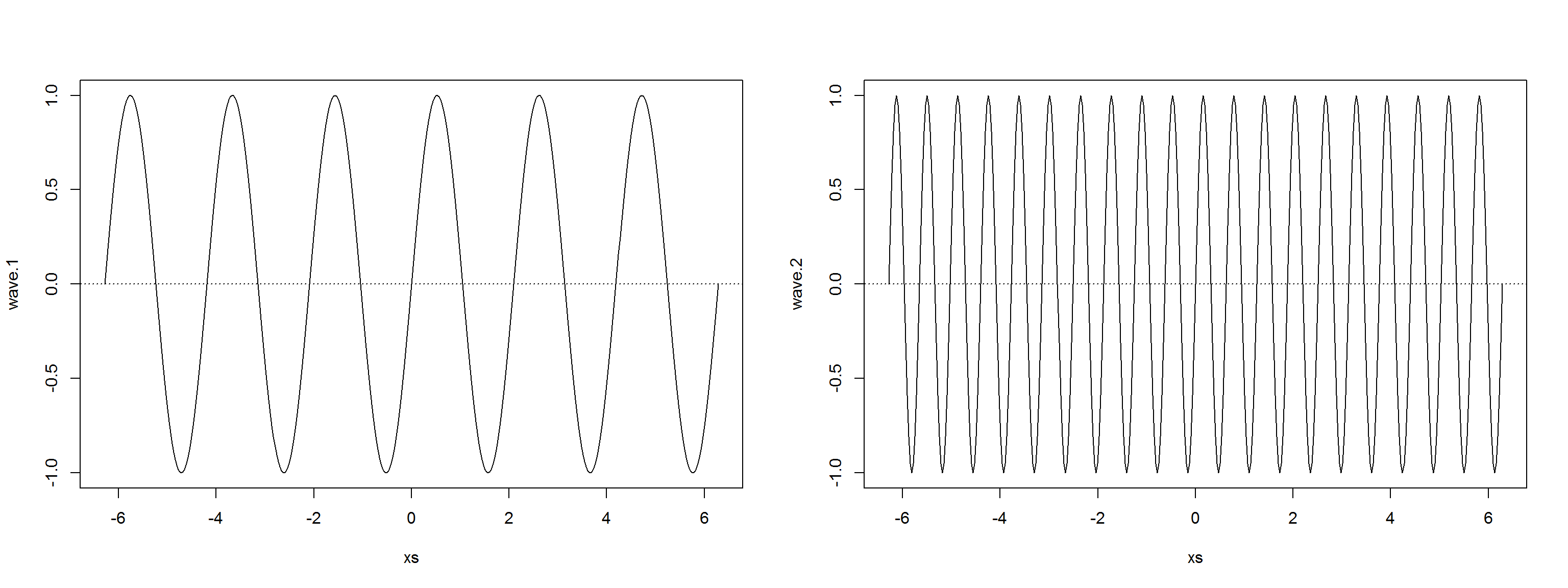which can be linearly combined into a complex wave:

wave.3 <- 0.5 * wave.1 + 0.25 * wave.2
plot(xs,wave.3,type="l"); title("Eg complex wave"); abline(h=0,lty=3)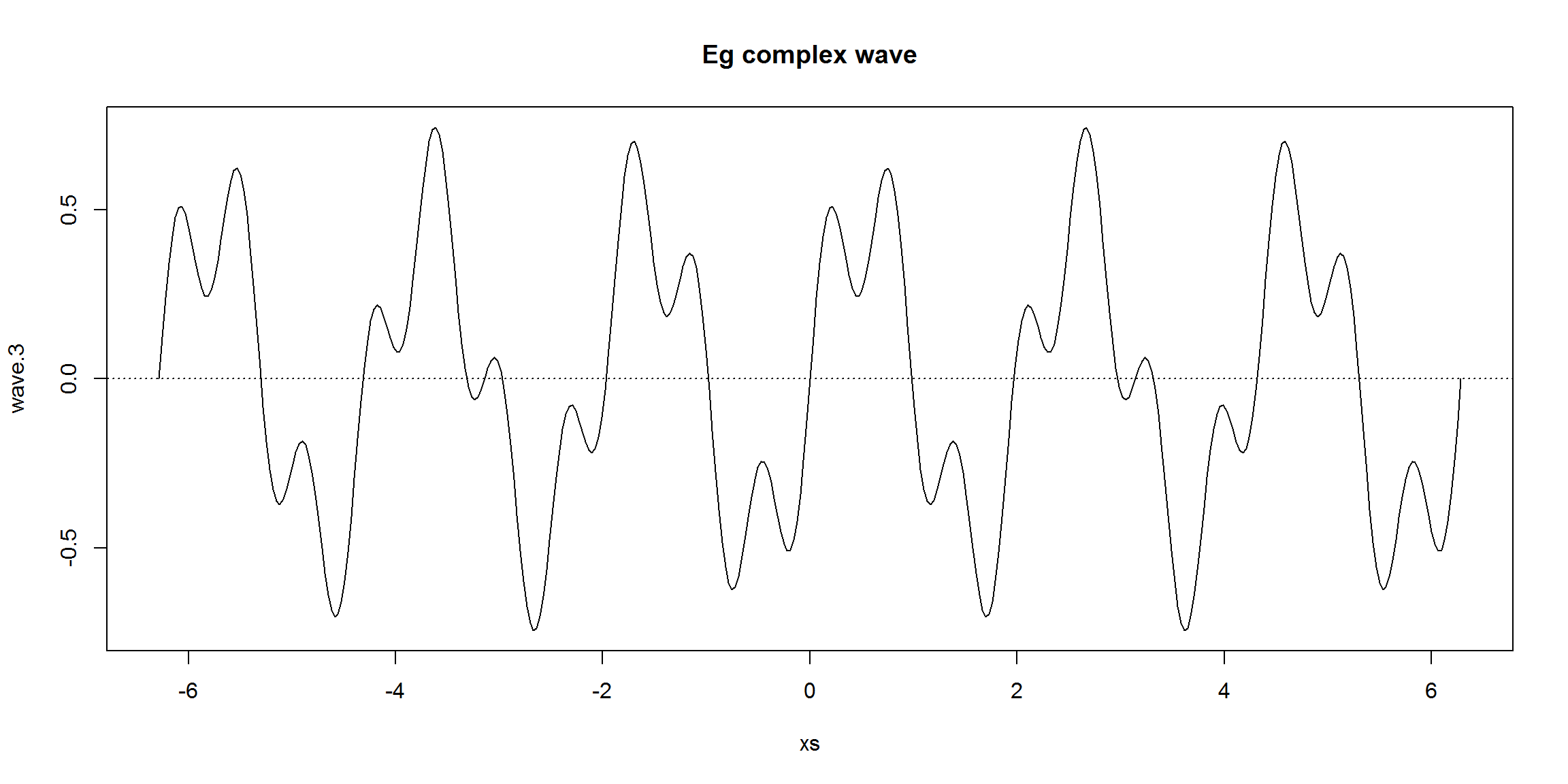## Fourier Series

Joseph Fourier showed that any periodic wave can be represented by a sum of simple sine waves. This sum is called the Fourier Series. The Fourier Series only holds while the system is linear. If there is, eg, some overflow effect (a threshold where the output remains the same no matter how much input is given), a non-linear effect enters the picture, breaking the sinusoidal wave and the superposition principle.

wave.4 <- wave.3
wave.4[wave.3>0.5] <- 0.5
plot(xs,wave.4,type="l",ylim=c(-1.25,1.25)); title("overflowed, non-linear complex wave"); abline(h=0,lty=3)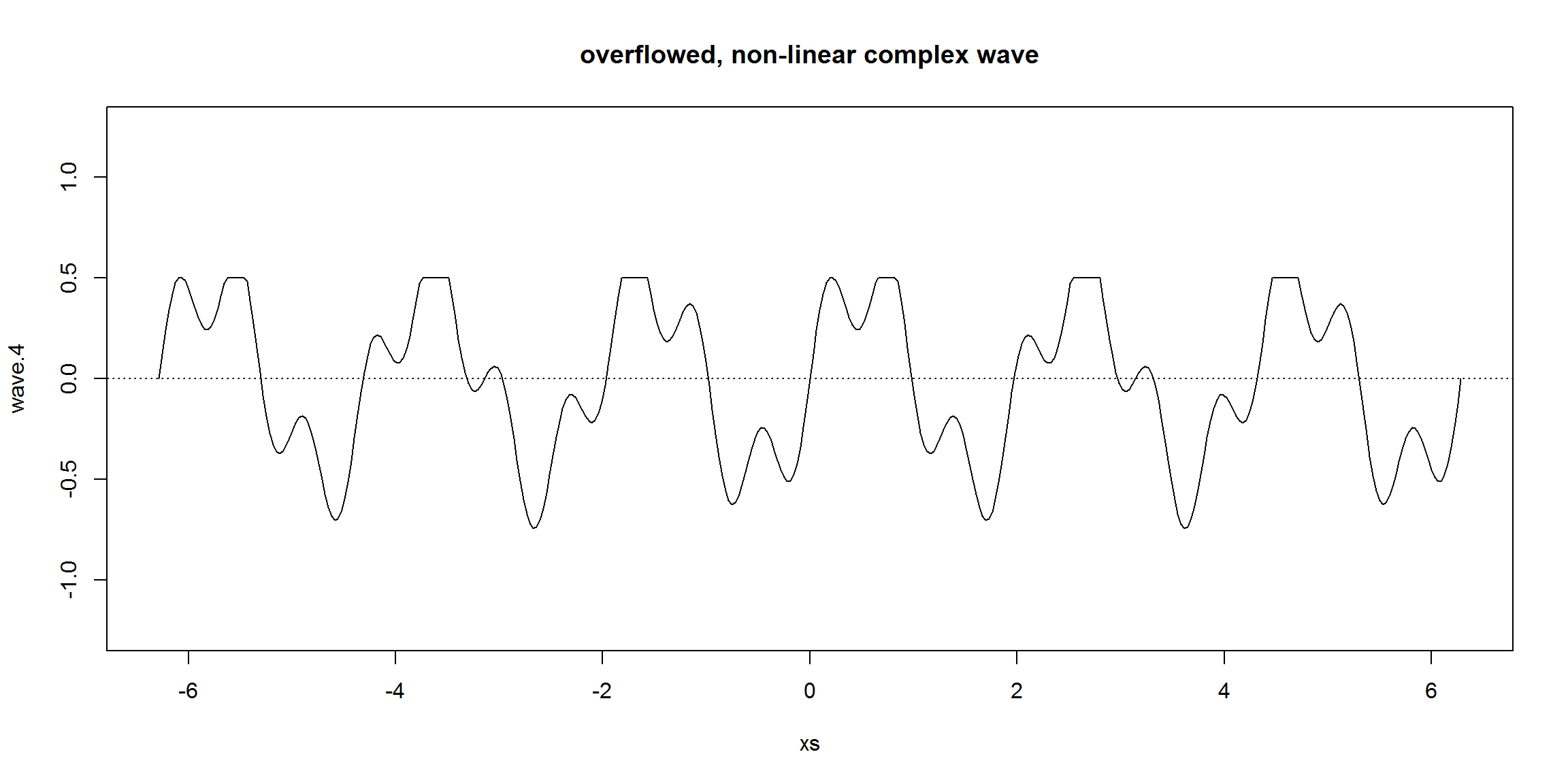Also, the Fourier Series only holds if the waves are periodic, ie, they have a repeating pattern (non periodic waves are dealt by the Fourier Transform, see below). A periodic wave has a frequency $$f$$ and a wavelength $$\lambda$$ (a wavelength is the distance in the medium between the beginning and end of a cycle, $$\lambda = v/f_0$$, where $$v$$ is the wave velocity) that are defined by the repeating pattern. A non-periodic wave does not have a frequency or wavelength.

Some concepts:

• The fundamental period, T, is the period of all the samples taken, the time between the first sample and the last
• The sampling rate, sr, is the number of samples taken over a time period (aka acquisition frequency). For simplicity we will make the time interval between samples equal. This time interval is called the sample interval, si, which is the fundamental period time divided by the number of samples $$N$$. So, $$si = \frac{T}{N}$$
• The fundamental frequency, $$f_0$$, which is $$\frac{1}{T}$$. The fundamental frequency is the frequency of the repeating pattern or how long the wavelength is. In the previous waves, the fundamental frequency was $$\frac{1}{2\pi}$$. The frequencies of the wave components must be integer multiples of the fundamental frequency. $$f_0$$ is called the first harmonic, the second harmonic is $$2*f_0$$, the third is $$3*f_0$$, etc.
repeat.xs     <- seq(-2*pi,0,pi/100)
wave.3.repeat <- 0.5*sin(3*repeat.xs) + 0.25*sin(10*repeat.xs)
plot(xs,wave.3,type="l"); title("Repeating pattern")
points(repeat.xs,wave.3.repeat,type="l",col="red"); abline(h=0,v=c(-2*pi,0),lty=3)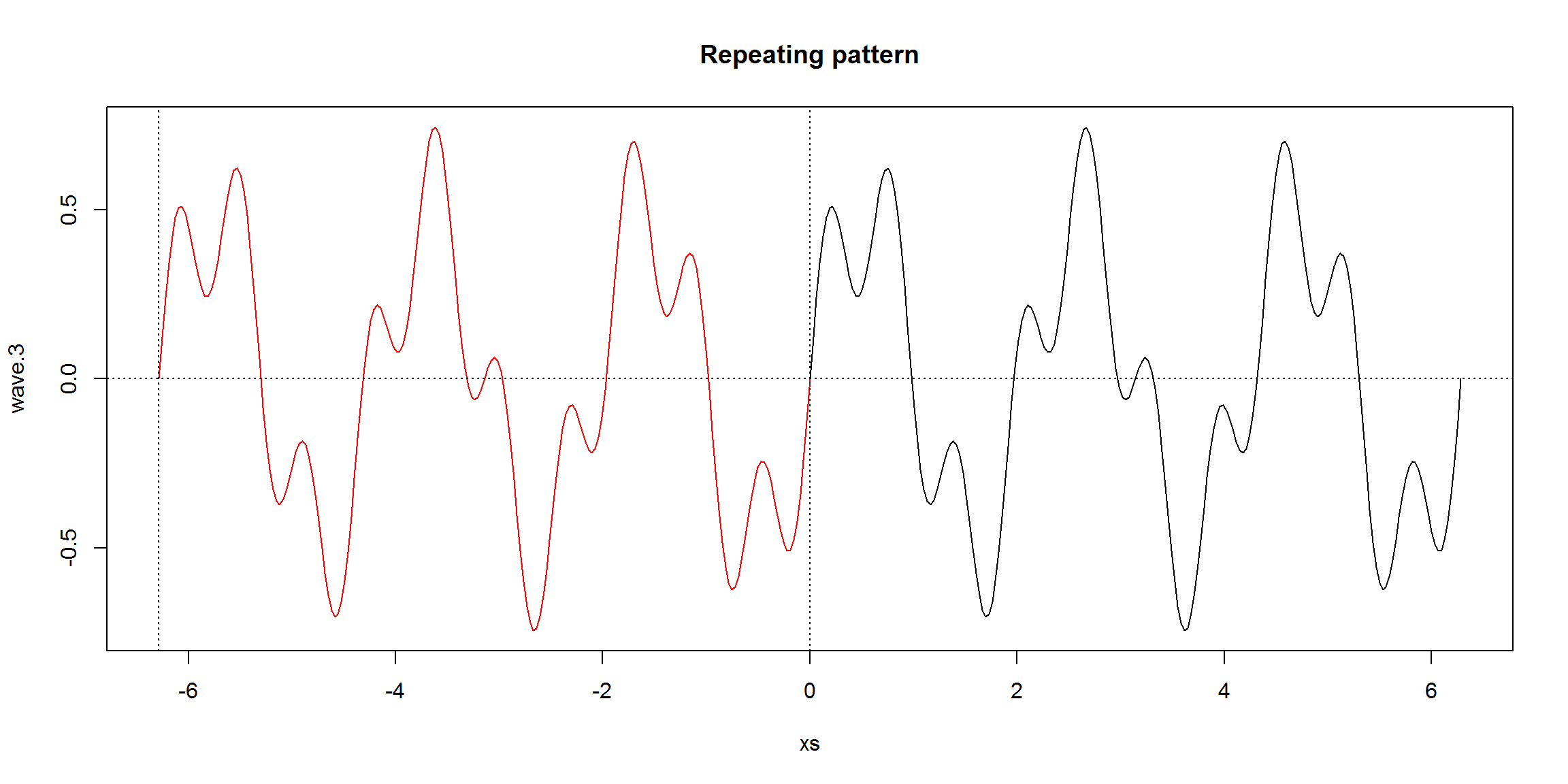wave.3 is the weighted sum of wave.1 and wave.2. This equation is the Fourier Series for wave.3:

$f(t) = 0.5 \times sin(3wt) + 0.25 \times sin(10wt)$

where $$w$$ is the angular frequency (aka angular speed) in radians/second, $$w=2\pi f_0$$, where $$f_0$$ is the fundamental frequency of the complex wave. In this case, the first component (wave.1) has thrice the frequency and the second component has 10 times that of $$f_0$$.

Here’s a R function for plotting trajectories given a fourier series:

plot.fourier <- function(fourier.series, f.0, ts) {
w <- 2*pi*f.0
trajectory <- sapply(ts, function(t) fourier.series(t,w))
plot(ts, trajectory, type="l", xlab="time", ylab="f(t)"); abline(h=0,lty=3)
}

# An eg
plot.fourier(function(t,w) {sin(w*t)}, 1, ts=seq(0,1,1/100))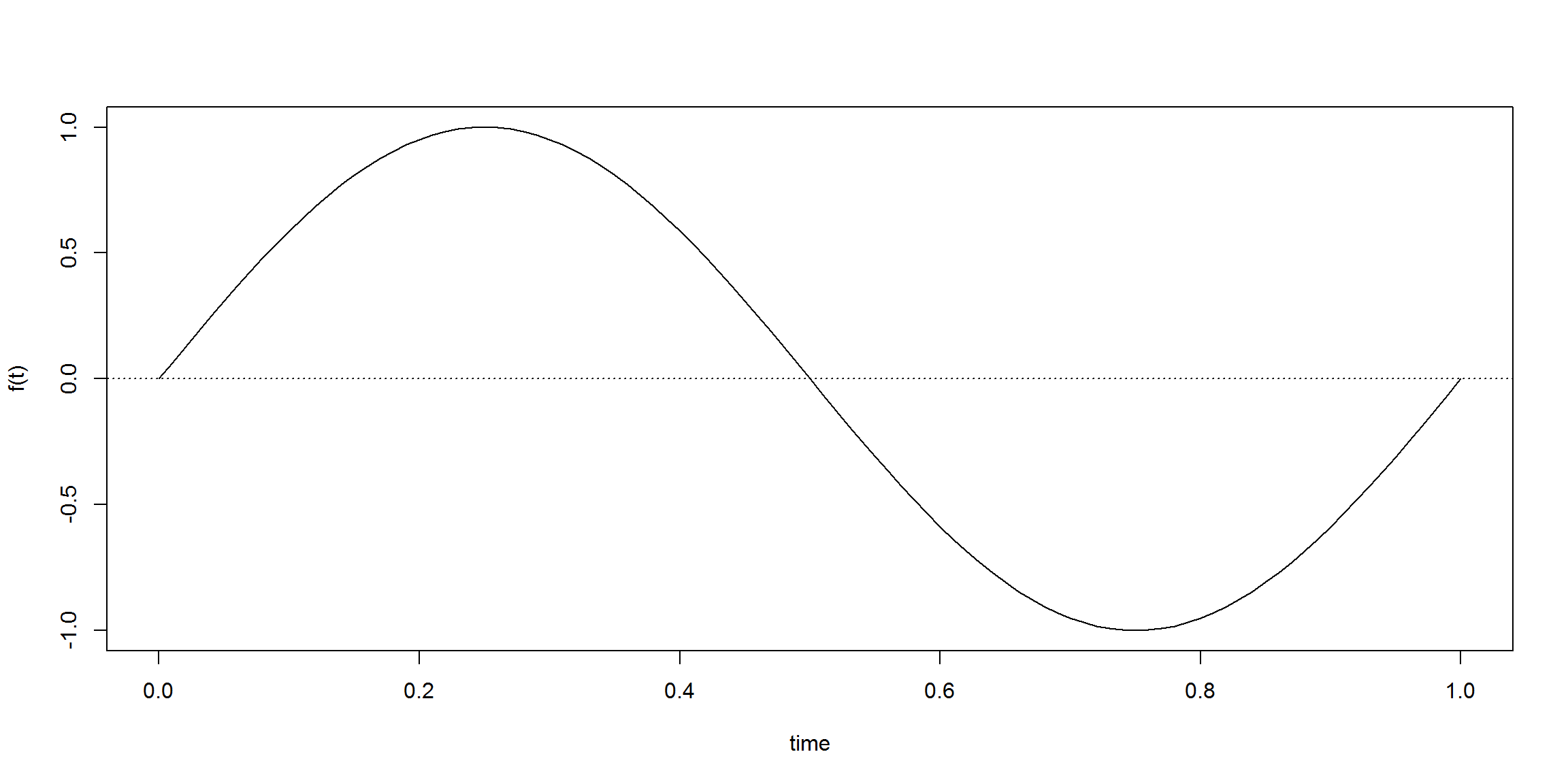And the plotting of equation $$f(t) = 0.5 \times sin(3wt) + 0.25 \times sin(10wt)$$:

acq.freq <- 100                    # data acquisition frequency (Hz)
time     <- 6                      # measuring time interval (seconds)
ts       <- seq(0,time,1/acq.freq) # vector of sampling time-points (s)
f.0      <- 1/time                 # fundamental frequency (Hz)

dc.component       <- 0
component.freqs    <- c(3,10)      # frequency of signal components (Hz)
component.delay    <- c(0,0)       # delay of signal components (radians)
component.strength <- c(.5,.25)    # strength of signal components

f <- function(t,w) {
dc.component +
sum( component.strength * sin(component.freqs*w*t + component.delay))
}

plot.fourier(f,f.0,ts)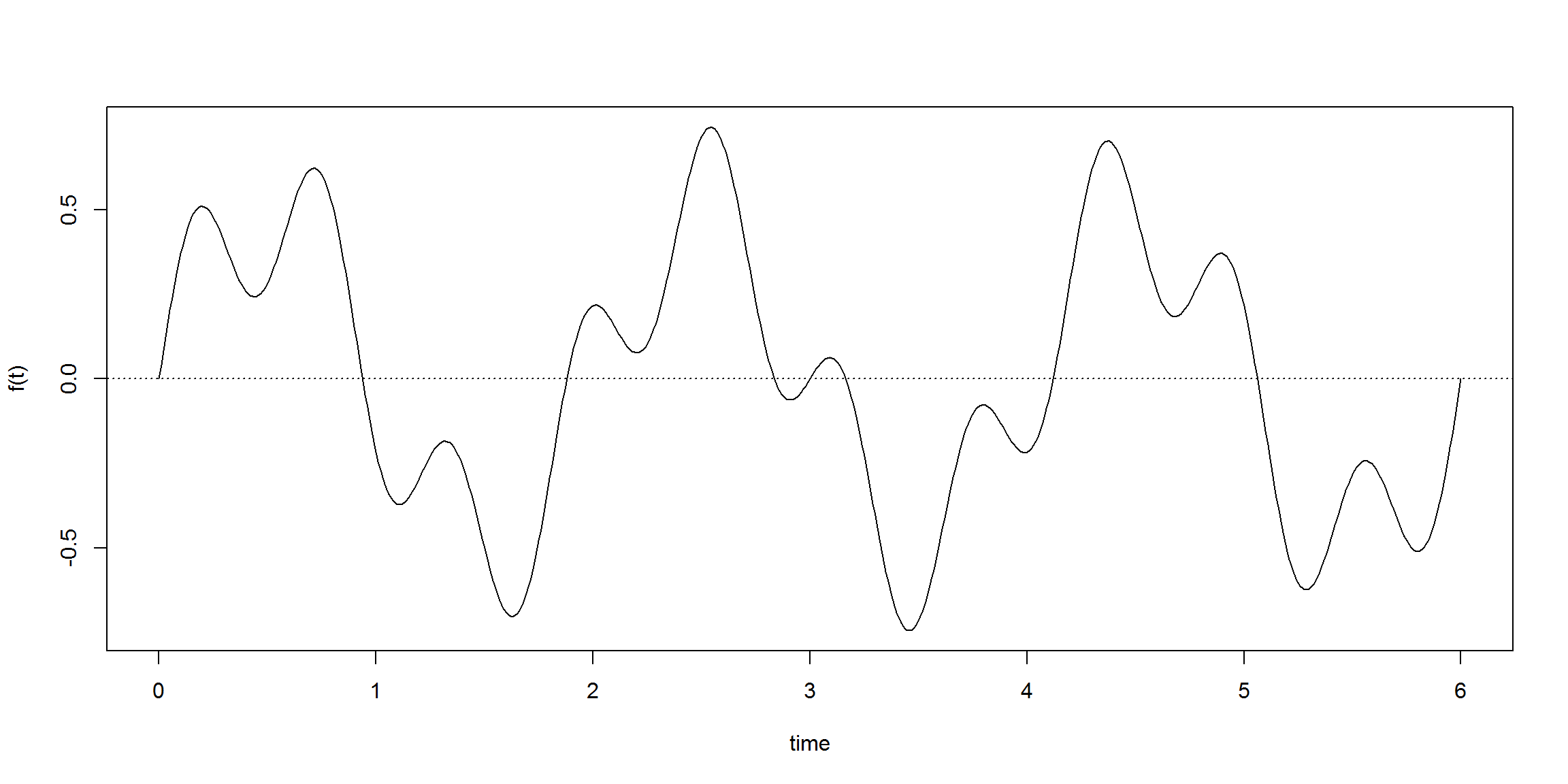## Phase Shifts

Another feature of the fourier series is phase shift. Phase shifts are translations in the x-axis for a given wave component. These shifts are measured in angles (radians).

Taking the previous example and shifting wave.1 by $$\frac{\pi}{2}$$ we would produce the following fourier series:

$f(t) = 0.5 \times sin(3wt + \frac{\pi}{2}) + 0.25 \times sin(10wt)$

which produces the following trajectory:

component.delay <- c(pi/2,0)       # delay of signal components (radians)
plot.fourier(f,f.0,ts)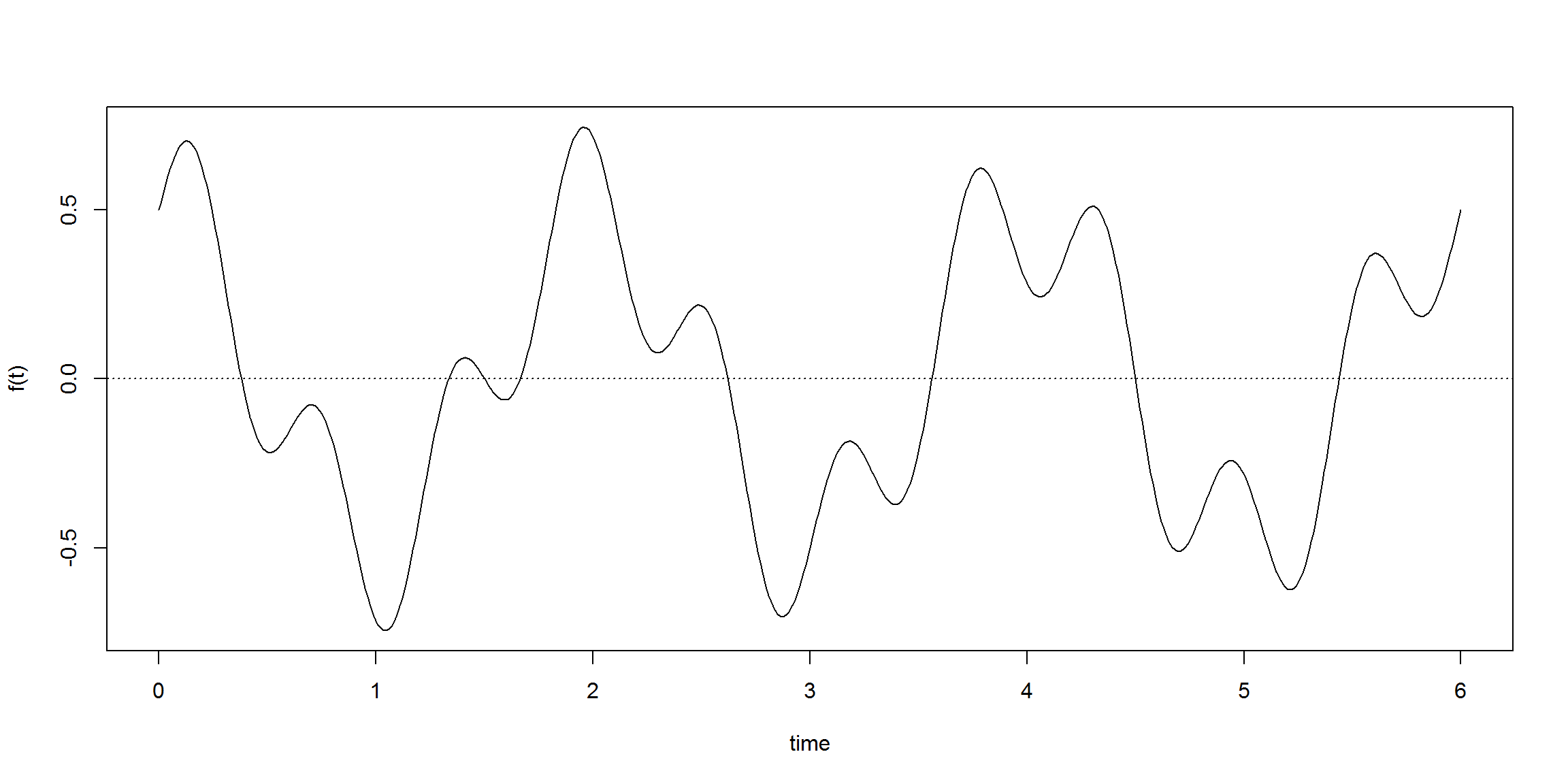## DC Components

This concept deals with translations over the y-axis. In this case corresponds to an additive constant signal.

Applying a DC component of $$-2$$ to the previous ware would result in the following equation and plot:

$f(t) = -2 + 0.5 \times sin(3wt + \frac{\pi}{2}) + 0.25 \times sin(10wt)$

dc.component <- -2
plot.fourier(f,f.0,ts)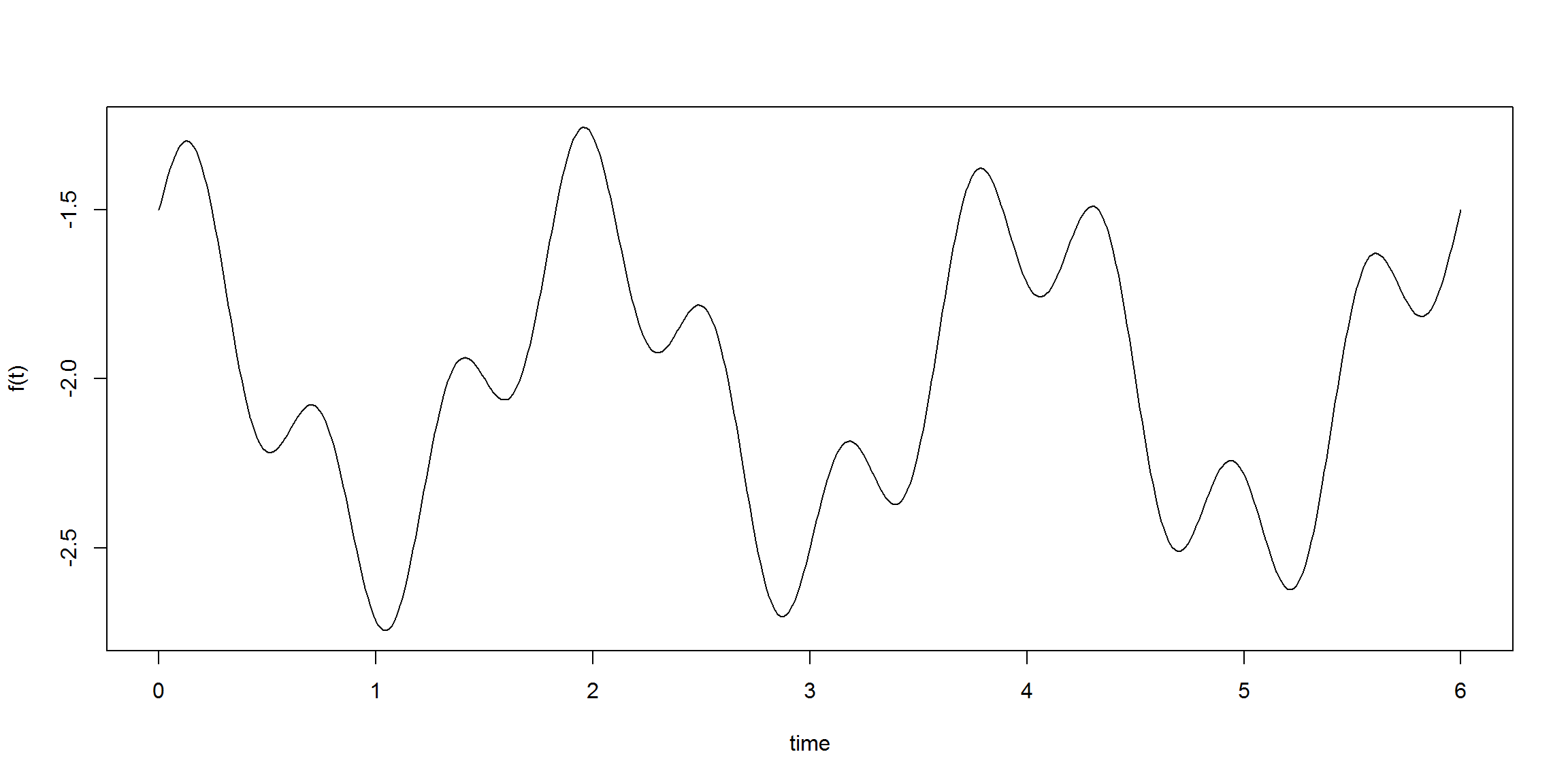## The General Equation

Adding these concepts we get the general form of the Fourier Series:

$f(t) = a_0 + \sum_k a_k \times sin(kwt + \rho_k)$

where $$a_0$$ is the DC component, $$w=2\pi f_0$$, where $$f_0$$ is the fundamental frequency of the original wave.

Each wave component $$a_k \times sin(kwt + \rho_k)$$ is also called a harmonic.

There is also an alternative representation using the identity:

$sin(a+b) = sin(a)cos(b) + cos(a)sin(b)$

which allow us to replace phase shifts with cosines.

The Fourier Transform (FT) is a generalization to solve for non-periodic waves. The FT assumes that the finite analyzed segment corresponds to one period of an infinitely extended periodic signal.

## Fourier Transform

The Fourier Transform sees every trajectory (aka time signal, aka signal) as a set of circular motions.

Given a trajectory the fourier transform (FT) breaks it into a set of related cycles that describes it. Each cycle has a strength, a delay and a speed. These cycles are easier to handle, ie, compare, modify, simplify, and if needed, they can be used to reconstruct the original trajectory.

The trajectory is processed thru a set of filters:

• each filter gives us a cycle and the remainder of the trajectory
• filters are independent, each one catches a different part of the trajectory
• there are enough filters to catch all of the trajectory, ie, the last filter leaves no trajectory remainder

The result cycles can be combined linearly, giving the same results no matter the mixing order.

So, the FT algorithm receives a trajectory, apply its filters to find the appropriate cycles, and outputs the full set of cyclic components. There are two algorithms:

• the Discrete Fourier Transform (DFT) which requires $$O(n^2)$$ operations (for $$n$$ samples)
• the Fast Fourier Transform (FFT) which requires $$O(n.log(n))$$ operations

This tutorial does not focus on the algorithms. There’s a R function called fft() that computes the FFT.

Here are two egs of use, a stationary and an increasing trajectory:

library(stats)
fft(c(1,1,1,1)) / 4  # to normalize
##  1+0i 0+0i 0+0i 0+0i
fft(1:4) / 4  
##   2.5+0.0i -0.5+0.5i -0.5+0.0i -0.5-0.5i

Soon we will be able to interpret these results :-)

First we need to recapitulate one particular mathematical point.

## Geometric Interpretation of Complex Numbers

Here’s a Geogebra applet to explain how we can interpret geometrically expressions like $$z \times e^{di}$$. Please try until you understand it:

<param name="ggbBase64" value="UEsDBBQACAgIALexbEIAAAAAAAAAAAAAAAAWAAAAZ2VvZ2VicmFfamF2YXNjcmlwdC5qc0srzUsuyczPU0hPT/LP88zLLNHQVKiuBQBQSwcI1je9uRkAAAAXAAAAUEsDBBQACAgIALexbEIAAAAAAAAAAAAAAAAMAAAAZ2VvZ2VicmEueG1szVnrctu4Ff6dfQoMf+zYjSUBIMBLVs6OnInbdJJMJt52Ov2xMxAJSVhThJakZNmzb9Mn6DP0f5+pBwBJ3XyTVutGsg0QOMDBuX3ngO7/uJxmaCGLUun83CNd7CGZJzpV+fjcm1ejTuT9+Pa7/ljqsRwWAo10MRXVuce61Futg6cuZWaxSs+9ISUkjAPeEUE66rCRP+wMBSMdxgkNeTSSYjTyEFqW6k2uP4upLGcikVfJRE7FR52Iyu45qarZm17v5uam23Dv6mLcG4+H3WWZeghOnpfnXt15A9ttLLrxLTnFmPT+8emj276j8rISeSI9ZKSaq7ffverfqDzVN+hGpdXk3At56KGJVOMJiBmwyEM9QzQDWWcyqdRClrB07dHKXE1nniUTuZl/5Xooa8XxUKoWKpWF0TLDMSeE0oj7fhAZhrpQMq9qWlLz7DW79RdK3rhtTc9yBKKFKtUwk8C9mINEKh8VoM1zbySyEp7L6jaTQ1E086vD+DCp7oAyZLCNk//cw2f4jGH76w6wxo15qNI6s7thxGP022+IYorRmWmIayg0QeCmsBvDvmuoa5hruKNhbjlzpMzRMEfD/DX5aoEeFrAeWElIGglj/jwB6e9i1yqUxNEmP/oAv+g44hGOV/w4PrM/9neHIwlenKW/l1a3vfQQjgF7hKNjcFyGEMrh0VkSEoPXkPgsIPxeriF+CTH7vQZ3+nXwo3JiaGu8qeS0NHDgxxYREEEcwj4IIYA5IjE0IYVhighHjMMjiVBg2hD5IUww5KMIGTriIxv3PII/zMyRAHHYywyG2DwDG4a4j4hFC4YAI5BFHEAf6gMF54jDIsOdGLZ+gFgAD36EGBzQYE1IzDisg2dgTpFPkG/WkhDRAAUUhQavCDMwFkTm7LApRQFGgVkKgAVg5YAKVkTIN9JAXM10qVrlTmQ2a61i9ajy2bza0F0yTZtupbeoU51cX7S6rmekKKt1MkgOqxTkksVGhnrVz8RQZpDHr4wjILQQmcEpy2Gk8wo1TkDd2LgQs4lKyitZVbCqRL+IhfgoKrm8BOqy4W1Z28TZl/MkU6kS+d/BS8wWZkPU5lFGVnmURTXnROsivbotwXXQ8p+y0AYySZcGhPH6GwBi37opylmXEUbD5guemwjj8zARr38ATm+bKd7F6x/uOMtFK5lYyrLR/rgw8VTr1Tx8KC90thqaaZVX78Ssmhe2JgKkL4xMg3ycSataa3OoLpLroV5eOZ36bq+fbmfwhN0BhuN3OtMFKoxcIOO4boeutTTmZC0VtjTYUuDGSCpt50lMLYVth661VGB1d7RaUtKISXDDRpUWR2Dzda+0LmNqlXmuqo/NQ6WS65Wkhv7zfDoEb6uXbW5JjrRlv7flX/1rWeQyc16UgyXnel46t25d81V/XsovopoM8vSrHENEfhEGFCvY2pGuTpzKRE1hoRuvVSeMWf8GR3WjqRwXspEws0WoU6ydxes+vTNst7os9PRDvvgJfGbrqP1eI0+/TAo1M66JhoDS13LlfakqBWB8ur4OhC9BisTgDSiyMkr8s9QgZKES9CEHWWeFdLUk0iP0Tk9nmVyiWsMeEvNqosF//qr/8y+NPstKAyMIdIMF6JMokglgK7iwCfFMTqEuRZV15Hw+lcCjNaqwJS+INa8lJ93YyW4sivTwF4CjVSnhFq0MAPMP+DoS2WwioNcltUeLW1lsaNfu9kmnDeeab2ZKbDRVgMQdCMKpWNqQrTHJXlFgJOgSP4wj0nxjQA9wgS4JGGsHIwM3I7VcMweoTd2B72060ircKgDQayjZS1tPVnX0285fVJrKvD2oyMH3rIUACmdOUgTwLV0EtUtnILnFnTW/qY3ypHmG2+bBXR7FmEUBBWCNcBR8Q8YKujTyCVyIcAiXRw5nc8a7Z3zXmJhT3uYPbo1JugHBQYzbb/SixowDn8Wc4YjFfkBxeIBxEz2dijxFuS3qbFyXYCJvVVAIfO4tBwC8YANy7t3arrPWvGoILty+9W47TmPzW2vvC+8ghzBV99g1Q9e0PoGf6RPrWFoas5qlxpAd27lzLyfcVdwc2STajZrGjW6h8MP6fKeKJJNbyrxwihTD8kSc7mgyeVyTgMtrwZc8pcm1nPWc2NpTj4+7Mb3fj6FYk/kCzqohW6Ilrl8I3eLaGnfNyJI0drkl9dAdWbMQREOhlmjQ0A8aqgE1uAyhCV2/3nXAms0GfE1S+WvuBChdnWBqWzVSyX4YmG5jIO0CrjPfpz6NzKsH/x4M3KhcviEIBK2Ba+psXsmrBOqSfPW2zB25RkaCcY2NPAzrMAps5wURkIc4BBR8JvBB5EONbTaw6lnuZ+W7XSvjb8euvLYjP8B8QW0+n72w+Q6pRpZQd5bmjXCj4wHcW5ew38kd+hNKdHmSnp4h0y9VDv1TbzMJ9Z7IToNdTN0w6TMg1d649gbV9curTU4dqHQxJYRCducAIiGzVsKAbBH2GY2Yz6ETRAdnrq2AuOvKn1X6tMbfNxoXZwjvq9/3R8hZhyl4J/ubm4TT6HGS/2NiXx5W9BxN6sOj77566Y8pl75quFBul0vvXbk0hIYaNW4XTO+/FzNd/rBPAdosOcwRj1CH7oQ66fIwDmNKAx/CPPKD+nqBOeeRzxgN/ZAydqxAlz+fiNdDdfq4Oezbp/utcemssab7DZv899+Pm8O+tWj1DNRP3SGbjHdY+BB8eK7dedv1jITXvOYpkjXrNHGYZfrmqxxlcmn1u/nCaM+rxKWzxmDHAHKfW4T8/RnvD7tEkP/zJSLe/Dx4o+jsf6XYSauVXFaa1rn1+1/nuvrB/UWvoZ55jeoHCN52OG2H1alr70vAZmNvi8sx69Y9LV5Woqi+GJhyog5AiBOISCgmumQHk3rrryLt/wXqf9S//R9QSwcI9PcfKoEIAABYIAAAUEsBAhQAFAAICAgAt7FsQtY3vbkZAAAAFwAAABYAAAAAAAAAAAAAAAAAAAAAAGdlb2dlYnJhX2phdmFzY3JpcHQuanNQSwECFAAUAAgICAC3sWxC9PcfKoEIAABYIAAADAAAAAAAAAAAAAAAAABdAAAAZ2VvZ2VicmEueG1sUEsFBgAAAAACAAIAfgAAABgJAAAAAA==" />
<param name="java_arguments" value="-Xmx1024m -Djnlp.packEnabled=true" />
<param name="cache_archive" value="geogebra.jar, geogebra_main.jar, geogebra_gui.jar, geogebra_cas.jar, geogebra_algos.jar, geogebra_export.jar, geogebra_javascript.jar, jlatexmath.jar, jlm_greek.jar, jlm_cyrillic.jar, geogebra_properties.jar" />
<param name="cache_version" value="4.2.24.0, 4.2.24.0, 4.2.24.0, 4.2.24.0, 4.2.24.0, 4.2.24.0, 4.2.24.0, 4.2.24.0, 4.2.24.0, 4.2.24.0, 4.2.24.0" />
<param name="showResetIcon" value="false" />
<param name="enableRightClick" value="false" />
<param name="errorDialogsActive" value="true" />
<param name="enableLabelDrags" value="false" />
<param name="showToolBar" value="false" />
<param name="showToolBarHelp" value="false" />
<param name="showAlgebraInput" value="false" />
<param name="useBrowserForJS" value="true" />
<param name="allowRescaling" value="true" />
This is a Java Applet created using GeoGebra from www.geogebra.org - it looks like you don’t have Java installed, please go to www.java.com

So when moving:

• the d slider makes the point moving in circles
• the z slider makes the circular wider or narrower

This makes expressions like $$z \times e^{di}$$ very good candidates to express circular motions.

To learn about using complex numbers in R check www.johnmyleswhite.com/notebook/2009/12/18/using-complex-numbers-in-r/

note: With this interpretation the great Euler’s formula, $$e^{\pi i} = -1$$, can be understood: it means a 180 degrees ($$\pi$$ radians) rotation, placing the point in the x-axis, in the opposite side of the unit circle, ie, at point (-1,0) in the complex plane, which is the integer $$-1$$.

## Cycle’s properties

We mentioned that each cycle has a strength, a delay and a speed. How can we represent them?

• The strength is represented by the circle size, which is controlled by $$z$$
• The delay, or starting point, is given by an initial value of $$d$$
• The speed will be represented by the rate of change of $$d$$ over time

Here’s an animation taken shamelessly from Better Explained describing a circular path:

Fiddle in the Cycles/Time textboxes to see what happens.

Anyway, remember this output?

fft(1:4) / 4  # to normalize
##   2.5+0.0i -0.5+0.5i -0.5+0.0i -0.5-0.5i

Here’s an animation for the same trajectory:

In the Cycles textbox the values after the colons mean the starting point of that cycle (in degrees), ie, the cycle’s delay. So :180 means that that cycle starts at the initial rotation of 180 degrees, or $$\pi$$ radians.

The cycles shown here for the trajectory 1,2,3,4 is 2.5 0.71:135 0.5:180 0.71:-135 which is just another way to represent the output of the fft() R function. The fft() function returns a sequence complex numbers, while the animation returns pairs strength:delay (in degrees).

Here’s a little function to convert the fft() output to the animation output:

# cs is the vector of complex points to convert
convert.fft <- function(cs, sample.rate=1) {
cs <- cs / length(cs) # normalize

distance.center <- function(c)signif( Mod(c),        4)
angle           <- function(c)signif( 180*Arg(c)/pi, 3)

df <- data.frame(cycle    = 0:(length(cs)-1),
freq     = 0:(length(cs)-1) * sample.rate / length(cs),
strength = sapply(cs, distance.center),
delay    = sapply(cs, angle))
df
}

convert.fft(fft(1:4))
##   cycle freq strength delay
## 1     0 0.00   2.5000     0
## 2     1 0.25   0.7071   135
## 3     2 0.50   0.5000   180
## 4     3 0.75   0.7071  -135

which is the output of the previous animation.

So this takes care of strength and delay. What about speed? That is given by the cycles’ sequence. The first cycle is stationary (0 Hz, ie, the DC component), then for every next cycle, the frequency increases (1Hz, 2Hz, 3Hz,…).

Try these cycle’s sequences in the animation:

• 1
• 0 1 this is the first animation
• 0 0 1
• and so on, until you understand the pattern…

A cycle sequence with just one 1 in the i-th position means a pure cycle of (i+1) Hz, ie, it completes i+1 cycles per time interval.

It’s also possible to combine! A sequence 1 2 3 means that the 0 Hz cycle has strength 1, the 1 Hz cycle has strength 2, and the 2 Hz cycle has strenght 3:

See how the biggest green vector at the left rotates faster? That one is the 2 Hz cycle that we gave the largest strength.

The yellow dots (or ticks) mean the equally spaced time intervals before the trajectory repeats itself. In this case there are 3 since we have cycles up to 2 Hz. At tick 0 the three cycles have a combine strength of 6 (1+2+3) since they all start at angle zero (no delays). At tick 1 their sum is -1.5 since both the 2nd and 3rd cycle make a negative contribution, which again happens at tick 2. After that, the trajectory restarts.

The blue line is the weighted sum of all the cycles’ values. Notice that the blue line also has values between the data points. Consider it as an interpolation, the values that this modeling suggests where the signal should be between two given data points. But the important part is that, at the required points, the function has the correct values.

With cycle set 1 2 3:180 we see that initially the 2 Hz cycle starts in the opposite side and so the combined strength of the first two cycles balance exactly the strength of the third cycle. That’s why the first trajectory number is zero.

Usually we want to know the inverse: what should the cycles be so that their combine strengths result on a given known trajectory? That is what DFT/FFT computes.

Say that we want values 4 0 0 0 in the time series (a peak every four units of time). We must have cycles that start initially with a sum of 4 and then cancel each other for the next 3 time steps. Use the animation to find what those cycles are. The solution is 1 1 1 1. That is, initially all four cycles contribute to the output, then at time t=1 the 2 Hz cycle cancels the 0 Hz cycle (the DC component) while both 1 Hz and 3 Hz are zero. For time t=2 see for yourself what cycles cancel each other. For the last three moments, there is a destructive interference between all four cycles (from 0 Hz to 3 Hz).

## The Equations

A Fourier Transform converts a wave from the time domain into the frequency domain. There is a set of sine waves that, when sumed together, are equal to any given wave. These sine waves each have a frequency and amplitude. A plot of frequency versus strength (amplitude) on an x-y graph of these sine wave components is a frequency spectrum (we’ll see one briefly). Ie, the trajectory can be translated to a set of frequency spikes.

In equation terms:

$X_k = \sum_{n=0}^{N-1} x_n e^{-i.2\pi k n/N}$

meaning:

• $$X_k$$ amount of frequency $$k$$ in the signal; each $$k^{th}$$ value is a complex number including strength (amplitute) and phase shift
• $$N$$ number of samples
• $$n$$ current sample, $$n \in \{0\ldots N-1\}$$
• $$k$$ current frequency, between 0 Hz to N-1 Hz
• $$1/N$$ not necessary but it gives the actual sizes of the time spikes
• $$n/N$$ is the percent of the time we’ve gone through
• $$2 \pi k$$ the speed in radians/second
• $$e^{-ix}$$ the backwards-moving circular path. This last three tell how far we’ve moved, for this speed and time.

The function fft() returns these $$X_k$$.

An inverse Fourier Transform (IFT) converts the frequency domain components back into the original time wave. This is given by the next equation:

$x_n = \frac{1}{N} \sum_{k=0}^{N-1} X_k e^{i.2\pi k n /N}$

A function that returns an interpolated trajectory given $$X_k$$. It is interpolated because the only data we know are the measures (eg: some points like (t=0,signal=4), (1,0), (2,0) and (3,0)). Everything else is given by the results from Fourier Series computed by fft().

To perform a IFT use fft(X.k, inverse=TRUE) / length(X.k).

Anyway, here’s a function that applies the previous equation, ie, makes the IFT:

# returns the x.n time series for a given time sequence (ts) and
# a vector with the amount of frequencies k in the signal (X.k)
get.trajectory <- function(X.k,ts,acq.freq) {

N   <- length(ts)
i   <- complex(real = 0, imaginary = 1)
x.n <- rep(0,N)           # create vector to keep the trajectory
ks  <- 0:(length(X.k)-1)

for(n in 0:(N-1)) {       # compute each time point x_n based on freqs X.k
x.n[n+1] <- sum(X.k * exp(i*2*pi*ks*n/N)) / N
}

x.n * acq.freq
}

Here’s two useful functions:

• plot.frequency.spectrum() plot a frequency spectrum of a given $$X_k$$
• plot.harmonic() plots the i-th harmonic on the current plot
plot.frequency.spectrum <- function(X.k, xlimits=c(0,length(X.k))) {
plot.data  <- cbind(0:(length(X.k)-1), Mod(X.k))

# TODO: why this scaling is necessary?
plot.data[2:length(X.k),2] <- 2*plot.data[2:length(X.k),2]

plot(plot.data, t="h", lwd=2, main="",
xlab="Frequency (Hz)", ylab="Strength",
xlim=xlimits, ylim=c(0,max(Mod(plot.data[,2]))))
}

# Plot the i-th harmonic
# Xk: the frequencies computed by the FFt
#  i: which harmonic
# ts: the sampling time points
# acq.freq: the acquisition rate
plot.harmonic <- function(Xk, i, ts, acq.freq, color="red") {
Xk.h <- rep(0,length(Xk))
Xk.h[i+1] <- Xk[i+1] # i-th harmonic
harmonic.trajectory <- get.trajectory(Xk.h, ts, acq.freq=acq.freq)
points(ts, harmonic.trajectory, type="l", col=color)
}

Let’s check that last eg. Notice that this plot is equal to the blue line in the animation:

X.k <- fft(c(4,0,0,0))                   # get amount of each frequency k

time     <- 4                            # measuring time interval (seconds)
acq.freq <- 100                          # data acquisition frequency (Hz)
ts  <- seq(0,time-1/acq.freq,1/acq.freq) # vector of sampling time-points (s)

x.n <- get.trajectory(X.k,ts,acq.freq)   # create time wave

plot(ts,x.n,type="l",ylim=c(-2,4),lwd=2)
abline(v=0:time,h=-2:4,lty=3); abline(h=0)

plot.harmonic(X.k,1,ts,acq.freq,"red")
plot.harmonic(X.k,2,ts,acq.freq,"green")
plot.harmonic(X.k,3,ts,acq.freq,"blue")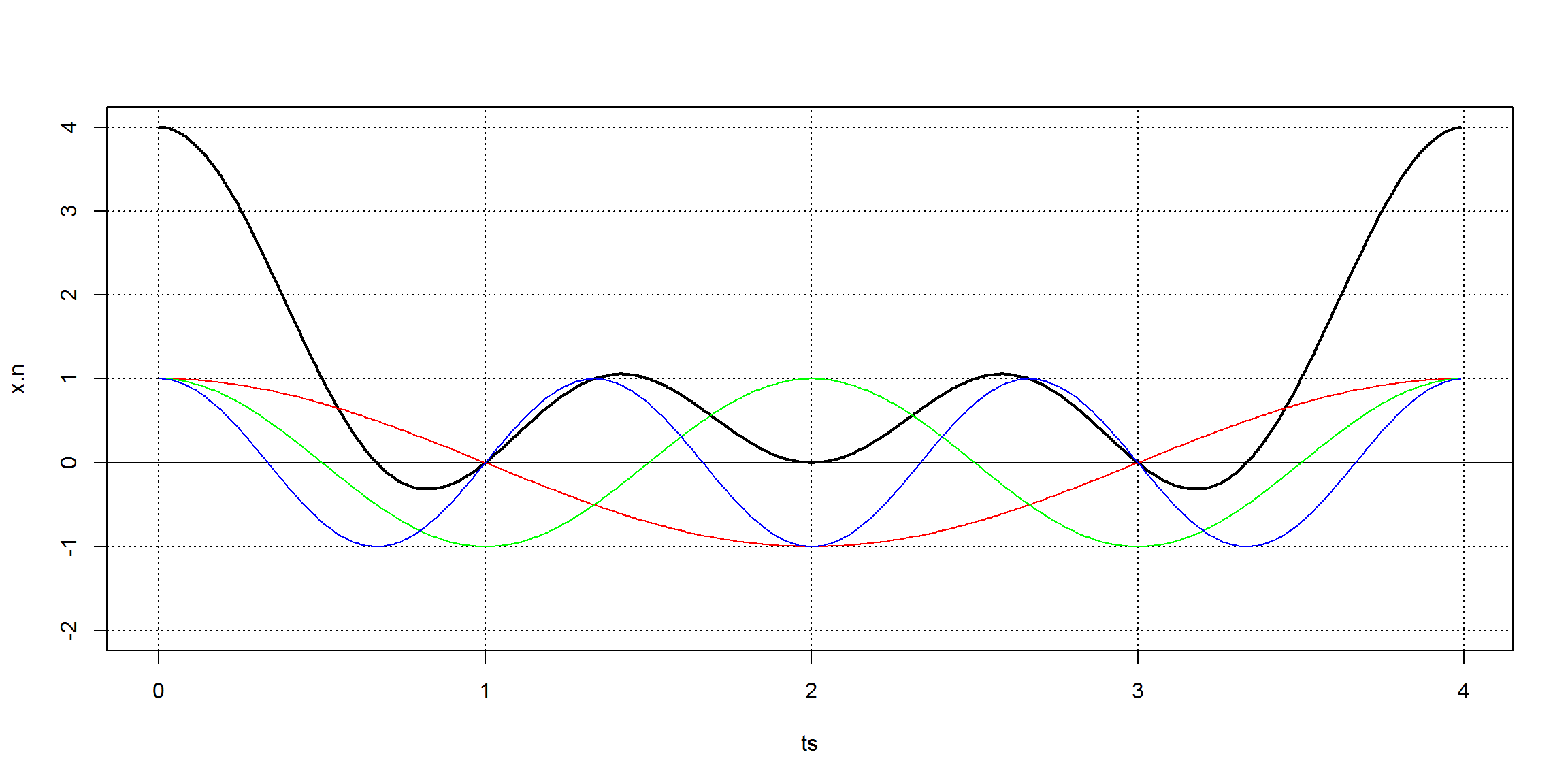The result has lots of harmonics. Some of them are possibly the result of noise, and hopefully will be very weak. Usually, we will just need the strong harmonics: those that contribute meaningfully to the signal.

The highest meaningful sin wave frequency – after the fft-analysis of the original waveform signal – is at half the data acquisition frequency, because our input signal is composed of real values (ie, trajectory has no imaginary parts). The last useful bin is at acq.freq/2. The Nyquist Frequency is half the sampling rate. The Nyquist frequency is the maximum frequency that can be detected for a given sampling rate. This is because in order to measure a wave you need at least one trough and one peak to identify it.

One important point is that any signal can be described in two ways:

• a time domain, the x-axis is a time variable and the y-axis the signal’s amplitude
• a frequency domain, the x-axis is a frequency variable and the y-axis the signal’s amplitude

Sometimes it’s easier to deal with one description, sometimes with the other.

The DFT and the IFT are the mathematical tools that translate between these two descriptions.

## Examples

Let’s try with one eg. We’ll make a trajectory given the following complex wave

$f(t) = 2 + 0.75 \times sin(3wt) + 0.25 \times sin(7wt) + 0.5 \times sin(10wt)$

acq.freq <- 100                    # data acquisition (sample) frequency (Hz)
time     <- 6                      # measuring time interval (seconds)
ts       <- seq(0,time-1/acq.freq,1/acq.freq) # vector of sampling time-points (s)
f.0 <- 1/time

dc.component <- 1
component.freqs <- c(3,7,10)        # frequency of signal components (Hz)
component.delay <- c(0,0,0)         # delay of signal components (radians)
component.strength <- c(1.5,.5,.75) # strength of signal components

f   <- function(t,w) {
dc.component +
sum( component.strength * sin(component.freqs*w*t + component.delay))
}

plot.fourier(f,f.0,ts=ts)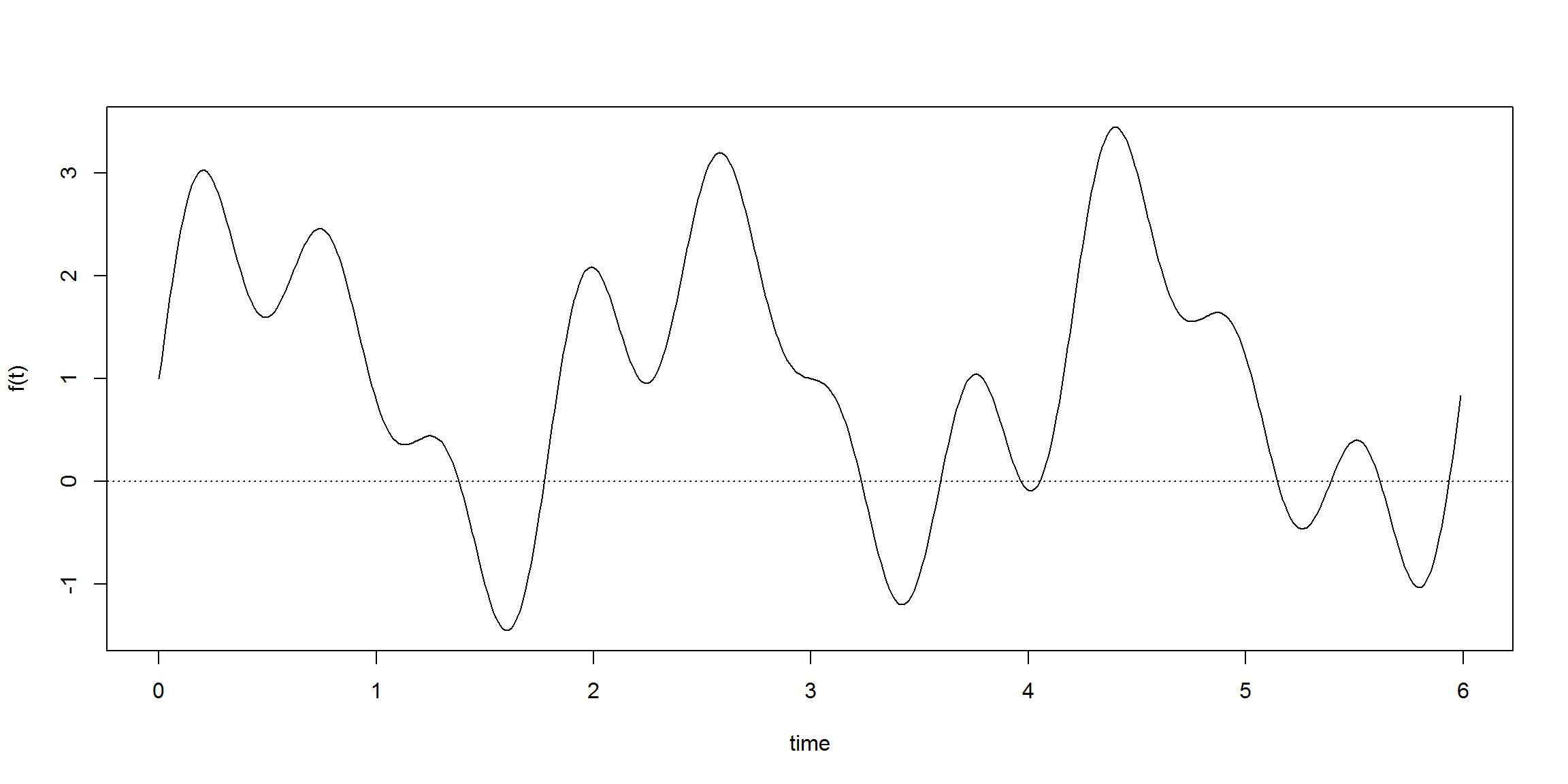Let’s assume that we don’t know the functional form of trajectory, we only have its contents, the period and the sampling time points:

w <- 2*pi*f.0
trajectory <- sapply(ts, function(t) f(t,w))
head(trajectory,n=30)
##   1.000000 1.162132 1.323161 1.481997 1.637568 1.788836 1.934801
##   2.074515 2.207089 2.331703 2.447610 2.554146 2.650736 2.736896
##  2.812242 2.876489 2.929454 2.971057 3.001324 3.020382 3.028458
##  3.025877 3.013056 2.990502 2.958803 2.918623 2.870694 2.815807
##  2.754805 2.688575

So, given that trajectory we can find where the frequency peaks are:

X.k <- fft(trajectory)                   # find all harmonics with fft()
plot.frequency.spectrum(X.k, xlimits=c(0,20))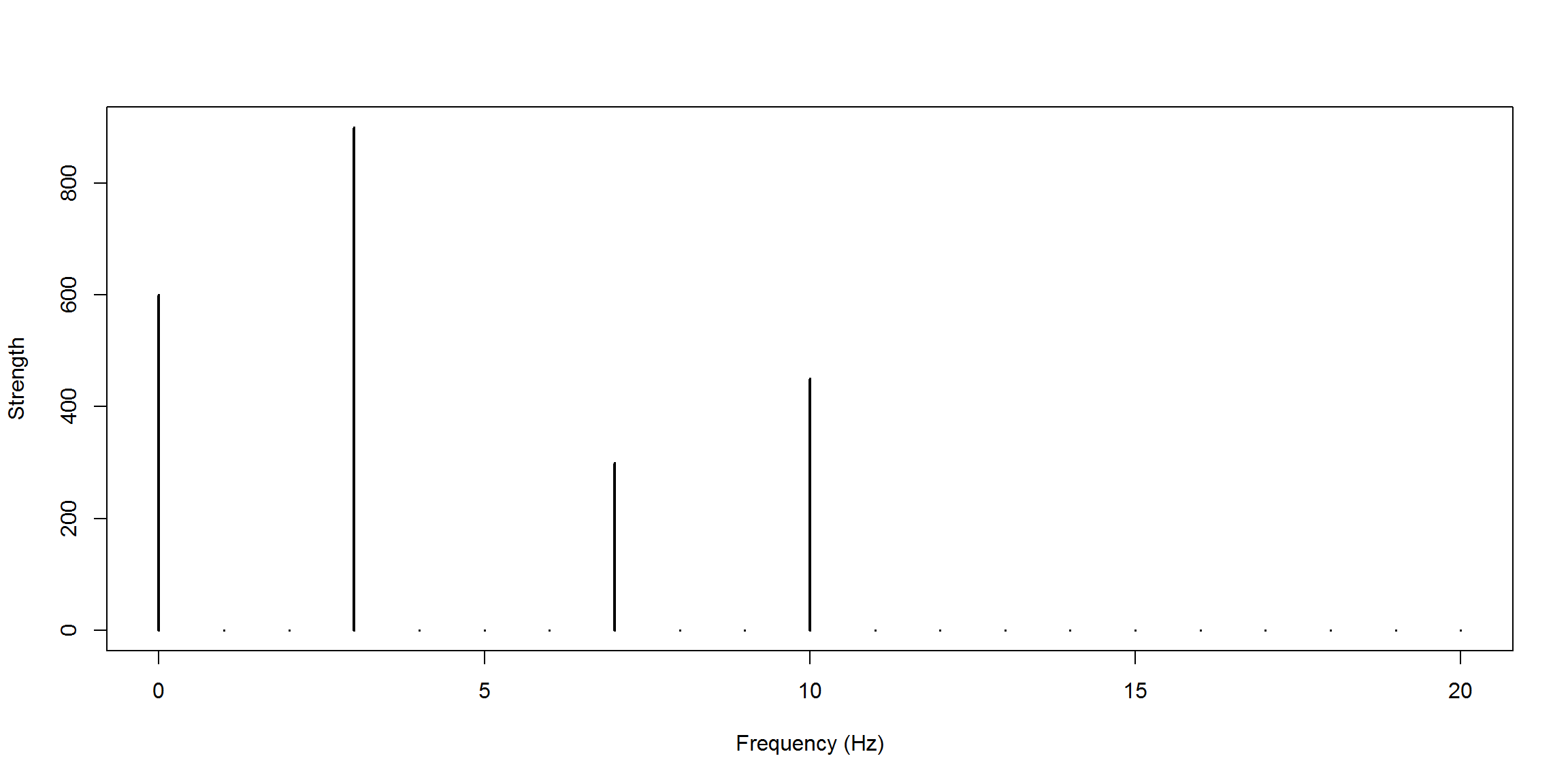And if we only had the frequency peaks we could rebuild the signal:

x.n <- get.trajectory(X.k,ts,acq.freq) / acq.freq  # TODO: why the scaling?
plot(ts,x.n, type="l"); abline(h=0,lty=3)
points(ts,trajectory,col="red",type="l") # compare with original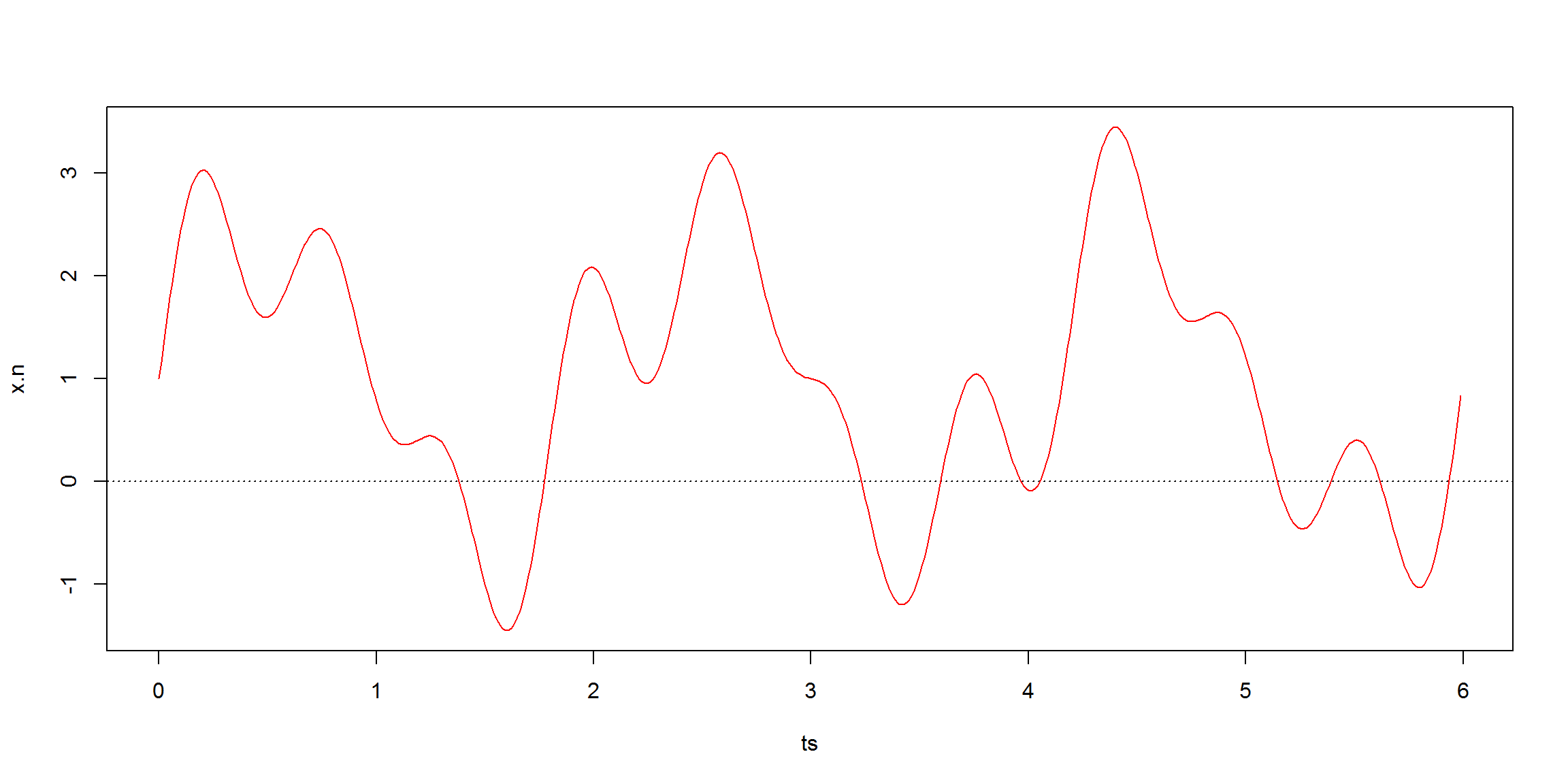This function is just for presentation purposes:

plot.show <- function(trajectory, time=1, harmonics=-1, plot.freq=FALSE) {

acq.freq <- length(trajectory)/time      # data acquisition frequency (Hz)
ts  <- seq(0,time-1/acq.freq,1/acq.freq) # vector of sampling time-points (s)

X.k <- fft(trajectory)
x.n <- get.trajectory(X.k,ts, acq.freq=acq.freq) / acq.freq

if (plot.freq)
plot.frequency.spectrum(X.k)

max.y <- ceiling(1.5*max(Mod(x.n)))

if (harmonics==-1) {
min.y <- floor(min(Mod(x.n)))-1
} else {
min.y <- ceiling(-1.5*max(Mod(x.n)))
}

plot(ts,x.n, type="l",ylim=c(min.y,max.y))
abline(h=min.y:max.y,v=0:time,lty=3)
points(ts,trajectory,pch=19,col="red")  # the data points we know

if (harmonics>-1) {
for(i in 0:length(harmonics)) {
plot.harmonic(X.k, harmonics[i], ts, acq.freq, color=i+1)
}
}
}

A decreasing signal:

trajectory <- 4:1
plot.show(trajectory, time=2)